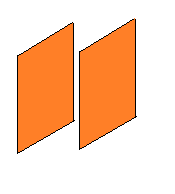# Force between plates of a capacitorWe have two parallel non-conducting square plates of side $l$ separated by a distance $l$ each plate having uniform charge density $\sigma$. Both the plates are fixed in their positions and are placed face to face as shown in the figure above.

Find the force exerted on one plate by the other one in Newtons. Submit your answer to 2 decimal places.

Details and Assumptions:

1) $l = 1 \text{ mm} , \sigma = 12.634 \times 10^{-3} \text{ C/m}^{2}$

2) ${\epsilon}_{0} = \text{ Permittivity of vaccum} = 8.85 \times 10^{-12}$

Extra Credit:

1) Find it's closed form, that is find the expression without putting the values.

2) Double credit : Solve for the closed form by hand. Without use of any computer.

3) Triple Credit-Post Solution. One who post complete solution wins my respect.

×

Problem Loading...

Note Loading...

Set Loading...